• 多行多列求和
万次阅读
2018-12-17 03:21:08

# 问题由来

前几天，一名网友在微信群里求助，说有一个表格汇总数据不会算。经过两天努力，我帮他写了一个宏，顺利完成了任务。这个按字段汇总的宏我以后再说，今天只说验算的公式。
由于源数据比较多，出于谨慎，我需要将宏算出来的汇总表进行验算，并且最好是用一个单元格用单个公式完成对源数据的加和运算，因此想编写一个公式把源数据表所有符合条件的数据加和，再对汇总表加和，比较两个和是否一致。

# 源数据格式

源数据是由上百个结构相同的数据块组成的数据块纵列。下面展示连续的两个数据块：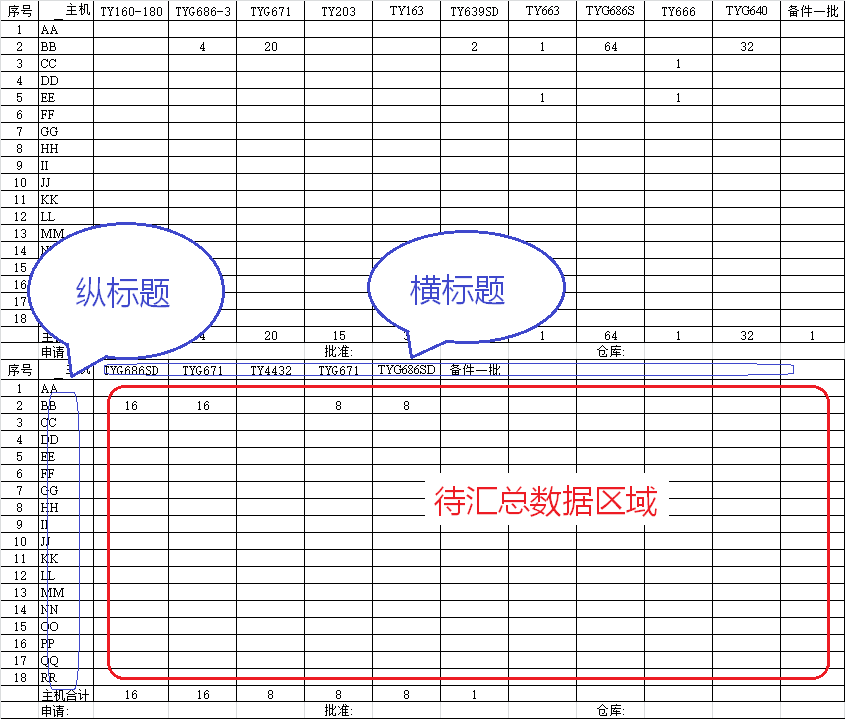每个数据块的格式如下：

1. 总共有21行、13列；
2. 第1行是标题，第20、21行是需要被忽略的数据，从第2行到第19行是需要汇总的数据；
3. 所有数据块的第二列是纵向标题，每块都一样，都是18个同样次序排列的字母；
4. 每块数据块的第一行是横向标题，此部分每个数据块都不一样，且数量有多有少；
5. 由此可以看出，需要求和每个数据块从第2行、第3列开始到第19行、第13列的数据块内的数据；

# 为什么不能直接求和

也许有人会说，直接求 C:M 的和不就行了？还真不行。每个数据块的第20行是一个合计行，不能被计算进总数内，所以不能直接求和。而这一个合计行的业务逻辑比较奇怪，我没看出这一行数据和主体数据块的关系，至少不是主体数据块的纵向求和，否则可以用求 C:M 的和然后除以 2 的方式来求所需要的总和。

# 我的解决过程

## 用 SUMPRODUCT 函数的失败过程

这个公式需求看上去一点都不难，我不想用数组公式，用 SUMPRODUCT 函数看上去应该是一个非常理想的选择。于是我写了如下的公式：

=SUMPRODUCT(C:M,((MOD(ROW(C:M)-1,21)+1)>1)*((MOD(ROW(C:M)-1,21)+1)<20)) ​​​​


看上去很完美，用 C:M 指定汇总的列区域，用 ROW 求出单元格所在行的值后用两个 MOD 指出汇总的行区域。两个参数涉及区域都是 C:M，大小完全一样，符合 SUMPRODUCT 函数对各参数之间大小完全匹配的要求。
但是，输入之后 Excel 提示我 #VALUE!

## 分析错误

问题到底出在什么地方？
我先构造了一个小范围的公式，即只计算一个数据块，公式如下：

=SUMPRODUCT(C1:M21,((MOD(ROW(C1:M21)-1,21)+1)>1)*((MOD(ROW(C1:M21)-1,21)+1)<20)) ​​​​


然后用公式标签页的公式求值功能对公式的运算过程进行跟踪，发现问题出在 ROW 函数身上。
我原以为，因为数组涉及到 C:M 多列，所以按 SUMPRODUCT 函数的工作流程，应该把涉及到的每个单元格都先用 ROW 求行值，再用 MOD 函数取余以确定其在每个数据块中的位置，就是说会生成一个21行×11列的数组。但跟踪下来完全不是这样。ROW 函数偷懒了。
ROW 看到要计算的是范围是一个 C1:M21 的方块，并没有一个单元格一个单元格去老老实实地求行值，而是把 C1:M1，C2:M2……C21:M21 这21行作为21个计算单元，只计算了21次，得出了21个结果，而不是 21×11=231 个结果，这样当然就跟 C1:M21 不匹配了。

## 解决问题

用 SUMPRODUCT 似乎走进了死胡同，我只能回头考虑用 SUM 的数组公式。一试之下，发现 SUM 比 SUMPRODUCT 果然要聪明一点，能清楚地领会我的意思。我的公式如下：

{=SUM(((MOD(ROW(C:C)-1,21)+1)>1)*((MOD(ROW(C:C)-1,21)+1)<20)*IF(ISNUMBER(C:M),C:M,))}


用了基本和上面 SUMPRODUCT 里一样的条件设置，就把和求出来了，对行值的判断还仅仅用了 C 一列。不同的是多加了一个 ISNUMBER 的判断，因为 SUM 毕竟不是 SUMPRODUCT，遇到非数值数据不会自动转化为零。

## 用 SUMPRODUCT 解决问题

那用 SUMPRODUCT 真的不能写这个公式吗？非也，经过我脑洞大开的思索，我在原来的公式里多加了一个条件判断

C1:M1=C1:M1


公式于是变成了这样：

=SUMPRODUCT(C:M,((MOD(ROW(C:C)-1,21)+1)>1)*((MOD(ROW(C:C)-1,21)+1)<20)*(C1:M1=C1:M1))


看上去是完全的废话条件，因为一个数肯定等于自身。但这个可谓是神来之笔，因为这一个多加的条件强迫 SUMPRODUCT 把参数内的单元格认真对待，横向的每一个单元格兄弟都不能被忽略，不能被第一列单元格大哥的光芒所掩盖。这样，逗号两边的两个参数所代表的数组终于得到平等，可以共同参与运算了。

# 我在 CSDN 的第一篇博客

这是我在 CSDN 的第一篇博客，见笑见笑。

更多相关内容
• 如下图左侧是某公司的产品销售表多行多列，现在想要快速统计出各产品的销售量。 ​ 在I2单元格输入公式=SUMIF($A$2:$A$12,H2,$B$2:$B$12)+SUMIF($C$2:$C$12,H2,$D$2:$D$12)+SUMIF($E$2:$E$12,H2,$F$2:$F$12)...

如下图左侧是某公司的产品销售表多行多列，现在想要快速统计出各产品的销售量。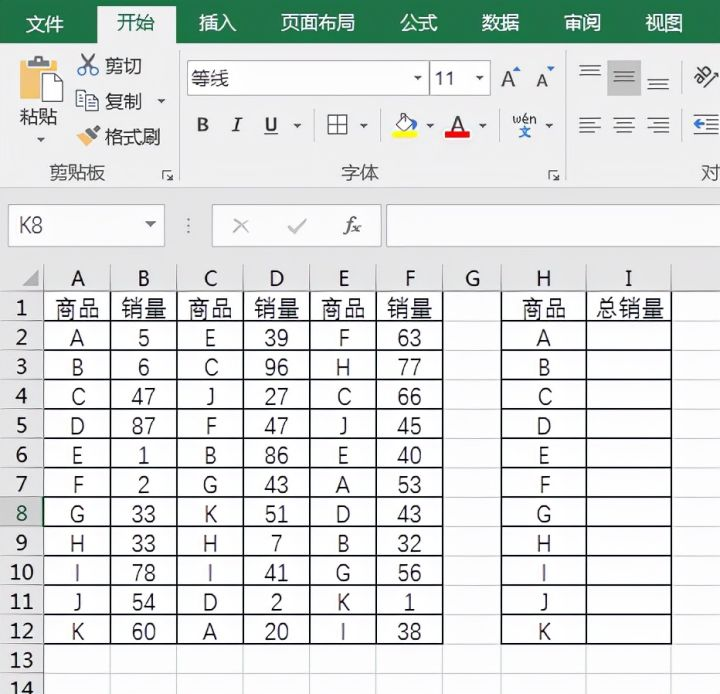在I2单元格输入公式=SUMIF($A$2:$A$12,H2,$B$2:$B$12)+SUMIF($C$2:$C$12,H2,$D$2:$D$12)+SUMIF($E$2:$E$12,H2,$F$2:$F$12)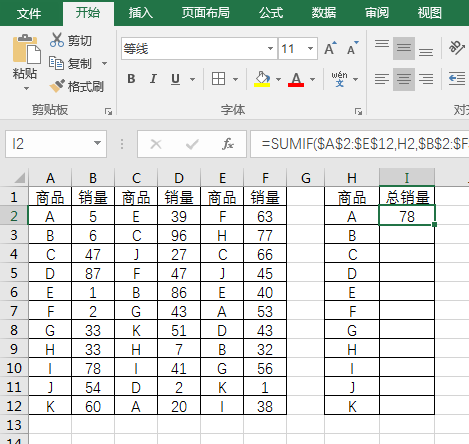然后将I2单元格公式下拉到底即可完成。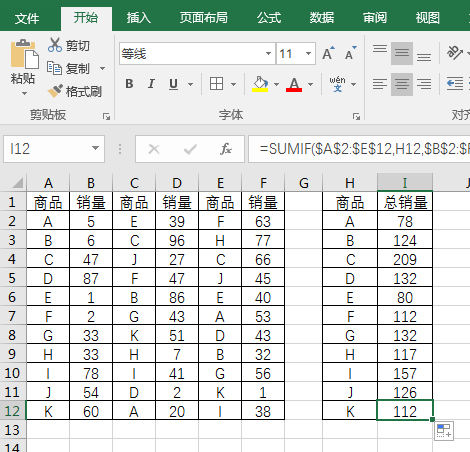下面跟大家介绍一下这个公式，SUMIF函数是Excel中的常用函数。使用 SUMIF 函数可以对报表范围中符合指定条件的值求和。sumif函数语法是：=SUMIF(range，criteria，sum_range)。我们将各区域A产品的销量计算出，然后将其相加即可得出总销售表中产品A的总销量。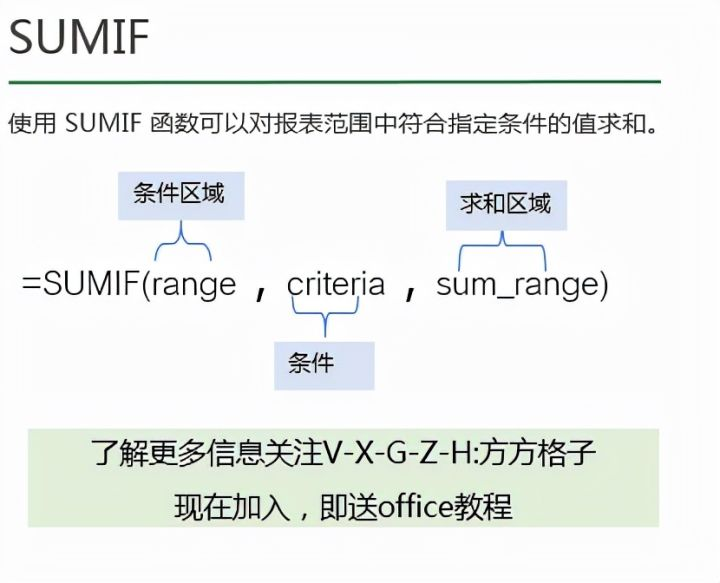如果觉得上一公式复杂我们也可以在I2单元格输入公式=SUMIF($A$2:$E$12,H2,$B$2:$F$12)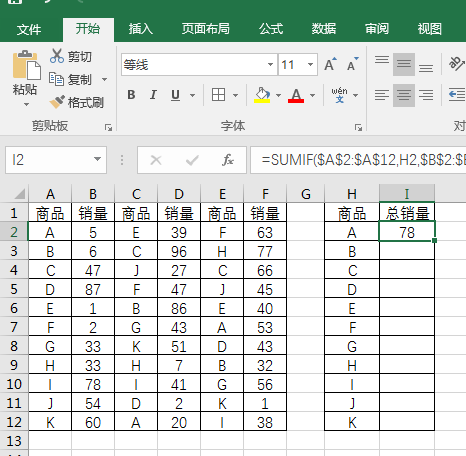然后将I2单元格公式下拉到底即可完成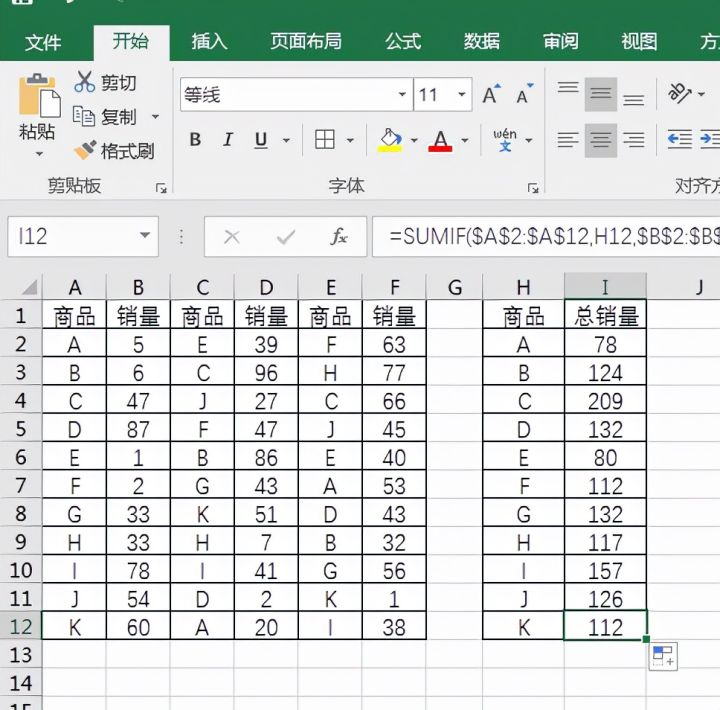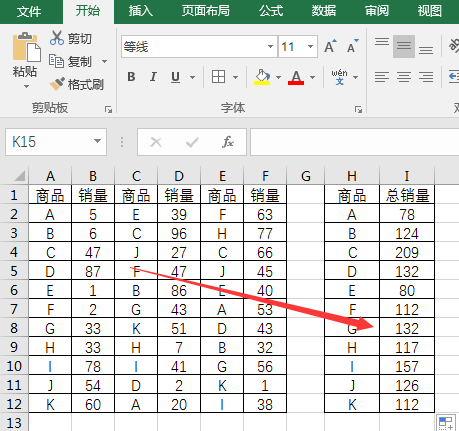展开全文• 他们每一个都各自专长：SUM——单行、单列、多行多列，无条件求和;SUMIF——行、列，一个条件求和;SUMIFS——行、列，多条件求和。根据上表可知，常用的三个求和函数，均无法满足所有的求和要求。是否有一个函数，...

在OFFICE官方函数列表中，常用的求和函数有三个：SUM,SUMIF,SUMIFS。

他们每一个都各自专长：

SUM——单行、单列、多行、多列，无条件求和;

SUMIF——行、列，一个条件求和;

SUMIFS——行、列，多条件求和。根据上表可知，常用的三个求和函数，均无法满足所有的求和要求。

是否有一个函数，可以满足所有的求和要求？

目前OFFICE最新版本为OFFICE 2019，尽管有新增函数，但并没有包含与求和有关的项目。那么有没有函数组合，满足多条件、多行或多列求和呢？

曾经在《如何快速解决多条件汇总难题——Excel中的三个简单方法》中，提到过数学集合的概念，并将其应用于多条件求和。

既然集合可用于多条件，那么也将其用于多行或多列求和。

实际上多行或多列，在Excel中称之为"区域"。因此只要能够使函数组合识别到数据"区域"，就可以进行计算。

与区域计算相关的函数常用的有两个，一个是SUMPRODUCT，一个是OFFSET。

下面就以统计最近一届奥运奖牌榜中国的金牌及银牌的总数为案例分别说明两个方法。方法一：SUMPRODUCT数组计算实现区域多条件求和观察SUMPRODUCT的参数是数据区域1、2……255。因此，可直接进行多区域计算，而计算条件，可作为区域参数即可，两者用符号"*"连接。

根据奖牌榜统计要求，包含一个求和区域及两个条件：

求和区域为"金牌"E、"银牌"F两列；

条件1是"最近一届"，也就是在年度A列选择最大的年份，这里使用MAX函数即可找到；

条件2是国家为"中国"。

将以上条件分别填入函数SUMPRODUCT中，公式为：

SUMPRODUCT((E2:F10)*(A2:A10=MAX(A2:A10))*(D2:D10="中国"))=44表哥TIPS:

SUMPRODUCT看似很好用，但由于参数个数的限制，最多不能超过255个，使用时需要注意。

方法二： OFFSET确定求和区域，加上SUMIFS多条件的特点。根据Excel官方说明中，OFFSET既可以返回一个单元格，也可以返回一片数据区域。其中前三个参数是必需项目。表哥Tips：

若第2、3两个参数为0，则可为空，但逗号不可省略。

同样以统计最近一届咱国家收获金牌和银牌数为例。这次我们使用

OFFSET来定义区域。由于所选区域为E、F两行，所以要用到数学中集合的概念。公式为：

OFFSET(E2:E10,,{0,1})公式中第三个参数的含义是，向右移动的列数。OFFSET中引入了集合{0,1}，则可理解为此公式包含两个区域：OFFSET(E2:E10,,0)和OFFSET(E2:E10,,0)。

确定了求和数据区域后，

结合SUMIFS函数的多条件的特点，再公式最外层加上SUM以进行集合运算。组合公式为：

SUM(SUMIFS(OFFSET(E2:E10,,{0,1}),A2:A10,MAX(A2:A10),D2:D10,"中国"))表哥Tips：

(1) 注意确认OFFSET返回区域是否在系统界内，超出系统极限值，会报错；

(2) SUMIFS的最多条件数是127，注意设置条件数在此范围内。

以上就是多行多列多条件求和的两种常用方法。

你学会了吗？

展开全文• 不同于之前写过的《Excel·VBA统计表生成函数及应用实例》对数据的2个条件汇总生成一个二维横纵统计表，仅对多行多列数据进行简单汇总，生成数据与原始数据格式一致 Sub 字典和数组简单汇总数据()..................

### 目录

不同于之前写过的 《Excel·VBA统计表生成函数及应用实例》对数据的2个条件汇总生成一个二维横纵统计表，仅对多行多列数据进行简单汇总，生成数据与原始数据格式一致

## 1，多行多列数据简单汇总

Sub 字典和数组简单汇总数据()
Dim arr, brr, dict, i, j, k
arr = [a1].CurrentRegion.Value
Set dict = CreateObject("scripting.dictionary")
ReDim brr(1 To UBound(arr), 1 To UBound(arr, 2))  '返回数组，定义为最大
For i = 2 To UBound(arr)
k = CStr(arr(i, 1)) & CStr(arr(i, 2)) '键
If Not dict.Exists(k) Then  '键不存在，新增
j = j + 1   '同时为brr数组序号和字典对应的值
dict(k) = j
brr(j, 1) = arr(i, 1): brr(j, 2) = arr(i, 2)  '赋值
brr(j, 3) = arr(i, 3): brr(j, 4) = arr(i, 4)
Else
r = dict(k)  '对应brr数组序号
brr(r, 3) = brr(r, 3) + arr(i, 3): brr(r, 4) = brr(r, 4) + arr(i, 4)  '累加
End If
Next
[g1].Resize(1, 4) = Array("产品名称", "型号", "数量", "金额")
[g2].Resize(j, UBound(brr, 2)) = brr  '仅赋值有写入brr的j行数据

End Sub


### 举例

左侧数据简单汇总C、D两列数据，得到右侧数据vba代码也可使用字典嵌套数组的形式汇总，但在输出结果时需要遍历字典item，较本例中直接将数组赋值给工作表，显得较为繁琐；且字典内部键值顺序也可能有所改变，较本例中与原数据顺序一致，也有所不足

## 2，数据汇总函数化、通用化

Function dictarr_summary(data_arr, col_arr)
'函数定义dictarr_summary(数组，汇总列号数组)对数组数据简单汇总，返回一个汇总后的二维数组
'data_arr为二维数组，col_arr为一维数组，注意汇总列号从1开始计数
Dim dict As Object, key_arr, result, i&, j&, arr, brr, k$, v&, r&, c Set dict = CreateObject("scripting.dictionary") '将data_arr排除col_arr中的数据，写入key_arr ReDim key_arr(1 To UBound(data_arr), 1 To UBound(data_arr, 2)) For j = 1 To UBound(data_arr, 2) index = Application.Match(j, col_arr, 0) '不在汇总列号数组中的，index是一个错误值 If TypeName(index) = "Error" Then For i = 1 To UBound(data_arr) key_arr(i, j) = data_arr(i, j) Next End If Next ReDim brr(1 To UBound(data_arr), 1 To UBound(data_arr, 2)) '临时数组，定义为最大 For i = 1 To UBound(data_arr) arr = Application.index(key_arr, i) k = CStr(Join(arr, "")) '键 If Not dict.Exists(k) Then '键不存在，新增 v = v + 1 '同时为brr数组序号和字典对应的值 dict(k) = v For j = 1 To UBound(data_arr, 2) brr(v, j) = data_arr(i, j) Next Else r = dict(k) '对应brr数组序号 For Each c In col_arr brr(r, c) = brr(r, c) + data_arr(i, c) Next End If Next If v = UBound(data_arr) Then dictarr_summary = brr Else ReDim result(1 To v, 1 To UBound(data_arr, 2)) '返回数组，避免无效部分 For i = 1 To v For j = 1 To UBound(data_arr, 2) result(i, j) = brr(i, j) Next Next dictarr_summary = result End If End Function  ### 举例1 对上面举例进行汇总，结果一致 Sub dictarr_summary测试() '多列汇总 Dim arr, brr, crr, result arr = [a1].CurrentRegion.Value brr = [a1].Offset(1, 0).Resize(UBound(arr) - 1, UBound(arr, 2)).Value crr = Array(3, 4) result = dictarr_summary(brr, crr) [g1].Resize(1, UBound(arr, 2)) = Application.index(arr, 1) [g2].Resize(UBound(result), UBound(result, 2)) = result '单列汇总 ' arr = [a2:c14].Value ' brr = Array(3) ' result = dictarr_summary(arr, brr) ' [g11].Resize(UBound(result), UBound(result, 2)) = result End Sub  ### 举例2 《excel吧提问》，汇总2列数据 Sub 多列汇总() Dim arr, brr arr = [a1].CurrentRegion.Value brr = Array(3, 5) result = dictarr_summary(arr, brr) [g1].Resize(UBound(result), UBound(result, 2)) = result End Sub  汇总结果## 3，字典嵌套字典汇总数据 本函数支持2列条件多列数据形式的汇总，将2列条件作为字典嵌套字典的键，返回的结果是按照第1个键的读入顺序，也可对dictarr_summary()函数结果排序获得一样的效果。（本函数字典嵌套2层字典的方式仅供参考） Function dict_summary(data_arr, col_key) '函数定义dict_summary(数组，汇总键列号数组)对数组数据按字典汇总，返回一个汇总后的二维数组 'data_arr为二维数组（从1开始计数），col_key为一维数组，键列号从1开始计数 '注意：键列号需指定顺序，仅支持2个 Dim dict As Object, col_sum, result, i&, j&, x&, y&, n& If UBound(col_key) - LBound(col_key) <> 1 Then Debug.Print "仅支持2个键": Exit Function Set dict = CreateObject("scripting.dictionary") '汇总列号，col_sum数组 ReDim col_sum(1 To UBound(data_arr, 2) - 2) For i = 1 To UBound(data_arr, 2) index = Application.Match(i, col_key, 0) If TypeName(index) = "Error" Then x = x + 1: col_sum(x) = i End If Next '仅支持字典嵌套1层键字典，然后嵌套1层汇总数据 For i = 1 To UBound(data_arr) k1 = data_arr(i, col_key(LBound(col_key))): k2 = data_arr(i, col_key(UBound(col_key))) If Not dict.Exists(k1) Then 'k1键 Set dict(k1) = CreateObject("scripting.dictionary") End If If Not dict(k1).Exists(k2) Then 'k2键 Set dict(k1)(k2) = CreateObject("scripting.dictionary") n = n + 1 '返回数组行数 End If For j = LBound(col_sum) To UBound(col_sum) '汇总键值 k3 = col_sum(j) dict(k1)(k2)(k3) = dict(k1)(k2)(k3) + data_arr(i, k3) Next Next ReDim result(1 To n, 1 To UBound(data_arr, 2)) keys1 = dict.keys: x = 0 For i = 0 To dict.count - 1 '遍历字典 keys2 = dict(keys1(i)).keys For j = 0 To dict(keys1(i)).count - 1 x = x + 1: result(x, 1) = keys1(i): result(x, 2) = keys2(j) '键赋值到数组 values3 = dict(keys1(i))(keys2(j)).Items For y = 0 To dict(keys1(i))(keys2(j)).count - 1 result(x, y + 3) = values3(y) Next Next Next dict_summary = result End Function  ### 举例 Sub dict_summary测试() Dim arr, brr arr = [a1].CurrentRegion.Value brr = Array(2, 1) '键有序 result = dict_summary(arr, brr) [f1].Resize(UBound(result), UBound(result, 2)) = result End Sub  汇总结果展开全文• ** SUMPRODUCT多列求和的应用，具体看图 ** • 有关多列条件求和对30列数据条件求和的快速SUMIF公式时间:2017-10-18作者:snow来源:Office之家今天和新手们分享有关条件求和的内容，高手请忽略。先来看一组销售数据，是某商场不同品牌电视机的三天销售记录：现在... • Sub 分厂家统计() Set cnn = CreateObject("adodb.Connection") Set rs = CreateObject("adodb.Recordset") cnn.Open "Provider = Microsoft.ace.Oledb.12.0;Extended Properties =TEXT;Data Source =" &... • mysql> select * from aa;+------+------+| id| name |+------+------+|1 | 10||1 | 20||1 | 20||2 | 20||3 | 200||3 | 500|+------+------+6 rows in set (0.00 sec)以id分组，把name字段的值打印在一行，逗号... • 由于这是多条件求和，所以要用到sumifs函数，又由于是多列求和，所以也要用到offset函数。 函数组合应用 =SUM(SUMIFS(OFFSET(C1:C25,,{0,1,2,3}),B1:B25,J5,G1:G25,"<>经理")) 这个应用的关键是offset函数... • /*NavicatMySQLDataTransferSourceServer:MySQLSourceServerVersion:50625SourceHost:127.0.0.1:3306SourceDatabase:db_bookTargetServerType:MYSQLTargetServerV... • ## shell awk 多列求和 千次阅读 2017-04-13 09:43:34 2016-04-12 14:05,5726,10085 2016-04-12 14:41,14628,17341 2016-04-12 14:42,19893,15488 2016-04-12 14:42,19893,7852 2016-04-12 14:43,20471,23041 这是格式化输出 如果不加\n换行，会把把结果输出到一...awk • <p>Let's say I have 5 different columns, <code>a, b, c, d, e, and I'm selecting multiple rows: <pre><code>$result = mysqli_query($conn,"SELECT a,b,c,d,e FROM posts WHERE submitter='$user'");...arrays mysql php
• EXCEL如何实现同条件多列求和就按正常方法求和就行了，不用管文字，系统能识别，我经常这样求和。excel中sumifs公式多列、或者区域求和1、首先在格中输入：=sumifs——回车——点击上面的【插入】标志：fx。2、求和...
• http://blog.csdn.net/rainyspring4540/article/details/50231435这里是oracle10g的环境数据库结构如下： 如果我想将相同名字的成绩显示在同一行，有2中基本展示：第一种展示(显示在同一行的不同上) 其sql如下：...
• 数据库表就是一个多行多列的表格。在创建表时，需要指定表的列数，以及列名称，列类型等信息。而不用指定表格的行数，行数是没有上限的。下面是tab_student表的结构： 当把表格创建好了之后，就可以向表格中添加...
• 一个行列式按指定的k行展开，指的是：先找出这k行的全部k阶子式，然后让这些k阶子式都乘以它们的代数余子式，再将所有的这些乘积求和，最后这个和就等于原来那个行列式。这就是行列式按k行进行拉普拉斯展开的定义。...
• 大家在日常工作中经常会遇到多列数据乘积后求和的问题，如图1所示，计算“数列1x数列2”后的总和，甚至需要计算“数列1x数列2x数列3”后的总和。图1我们一般会采用常规方法：先计算A2xB2xC2、A3xB3xC3……，在计算...
• '统计个excel表格的数据，每个行列值累加汇总到一个表格中 Sub huizong() '存放文件的目录 dirPath = "C:\Users\zhaigx\Desktop\1" fname = Dir(dirPath &amp; "\" &amp; "*....
• 目的：要将 prc_ben_type 列数据转为多列标题，并对其数据求和； set @acct_id = '001000001'; set @company_code = 'SZWX'; set @period_code = '201810'; set @city_codes = 'BJS,SHS,GUANGZS,SHENZS'; DROP ...
• 摘要:求和函数中，通常指使用sum对列进行聚合计算，下文讲述同时对多列多行采用sum计算，如下所示:实验环境:sqlserver 2008 R2---sum用法:select sum(表达式) from [表名] where [条件]---sum多列汇总求和select sum...
• 一个文件，两列，多行。第一是字母，第二是数字，同数据之间通过空格分割。统计首字母相同的第二之和。样例输入：A 5B 6OO 7A 6A 2OO 2输出：A：13B:6OO:9面试官要求30分钟内实现，python，C++实现。夸下5...
• 在求平均值时，既可一次只对一行一列或一个单元格区域求平均值，也可以一次对多行多列或多个单元格区域包括不连续的单元格区域批量快速求平均值；在批量求平均值时，需要用快捷键才能实现。一、Ex...
• 题目： 输入若干行，每行若干整数，求各行若干整数的和 要点： 空列表的插入 列表字符转整型 需增加异常处理 展示： n = list(map(int, input().split())) m=[] try: while True: ... ...python
• 本例的实现实质为两个步骤的求和：对于计算合计，利用For循环遍历每一数据，然后VBA中使用工作表函数SUM对同一的数据进行求和；对于计算行合计，利用For循环遍历每一个数据行，然后使用VBA中的工作表函数SUM对...
• 时间转化，按周，按日，按时，按年统计必备 select DATE_FORMAT(create_time,'%Y%u') weeks,count...and visitTime between "2016-12-16 11:11:06" and "2016-12-20 11:16:06" group by recordTime 更函数参考： ...
• st.name,c.NAME course,sc.score FROM students st LEFT JOIN scores sc ON st.id=sc.sid LEFT JOIN courses c ON sc.cid=c.id) t 对上表按人员id分组，并将分完组的个分数相加，组合到一行中，并对行求和： ...
• python pandas行、列求和及累加求和 data[‘合计’]=data.apply(lambda x: x.sum(),axis=1) #按列相加各行数 data.loc[‘小计’]=data.apply(lambda x: x.sum(),axis=0) #按行相加各列,增加小计， 要注意的是小计中...
• 本文介绍的是python中pandas.DataFrame对行与列求和及添加新行与列的相关资料，下面话不说，来看看详细的介绍吧。方法如下：导入模块：from pandas import DataFrameimport pandas as pdimport numpy as np生成...python对列求和
• 五、对表格或数组进行或列求和 在做报表时，经常会需要对取得的数据进行行或列的求和汇总操作，下面的代码可以完成对数组的行或列的求和操作，也适用于对格的指定区域进行求和汇总。 函数代码 Public Function ......